# University of Wisconsin Green Bay

A delivery truck with two stacked cartons in the back brakes quickly. The carton on the bottom just barely stays put on the floor of the truck. Does the top carton stay put or does it fall off? The top carton has a mass of 27 kg and the mass of the bottom carton is 22 kg. The coefficient of static friction between the bottom carton and the truck is 0.42, and the coefficient of kinetic friction for that surface is 0.35. The coefficient of static friction between the cartons is 0.40, and the coefficient of kinetic friction is 0.32.

• In this problem, you are asked to relate motion (of the cartons) to force (the friction between them). Force and motion of a single object are always related through Newton’s Second Law, so this is a force or 2nd Law problem.

In addition, note that you must treat the two cartons as separate systems. You don’t know if they move together as a single unit or not, and cannot answer that question without examining the forces between them. Therefore, you will need to draw a picture and set up equations for each carton individually.

•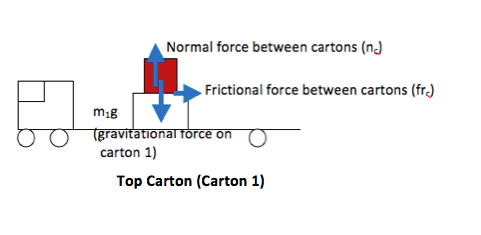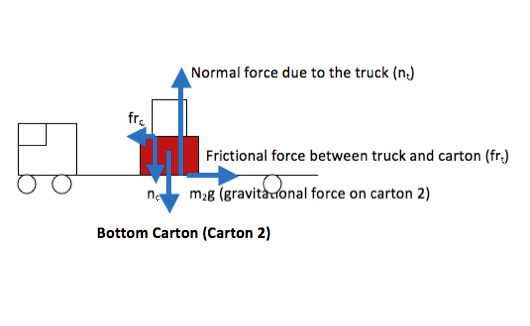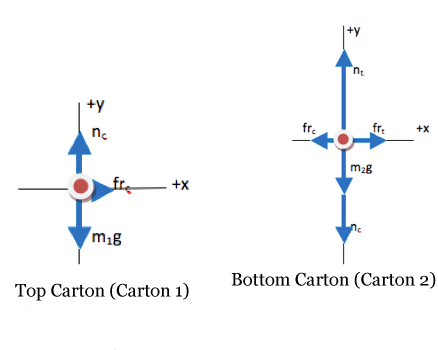•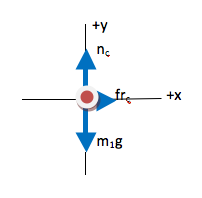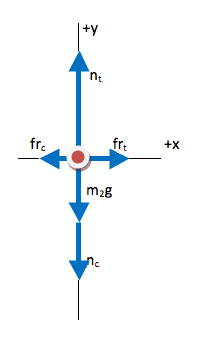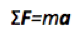The key equation for any problem that relates forces and motion is Newton’s Second Law. Regardless of what quantity you are asked to find, begin with the Second Law. If additional information is needed, it will become apparent as you proceed.

For multiple object problems, you will always need the additional information summarized as Newton’s Third Law (the interaction between two objects is felt by both objects equally and in the opposite direction.) In this example, that understanding has already been used—the contact force between the cartons was given the same symbol for each, and the frictional force between the cartons was given the same symbol for each. If you did not recognize the Third Law as you drew the FBDs, that is ok. When you start to solve the equations, you will find that you have too many unknowns and you can use the Third Law to reduce them at that point.

• Step 1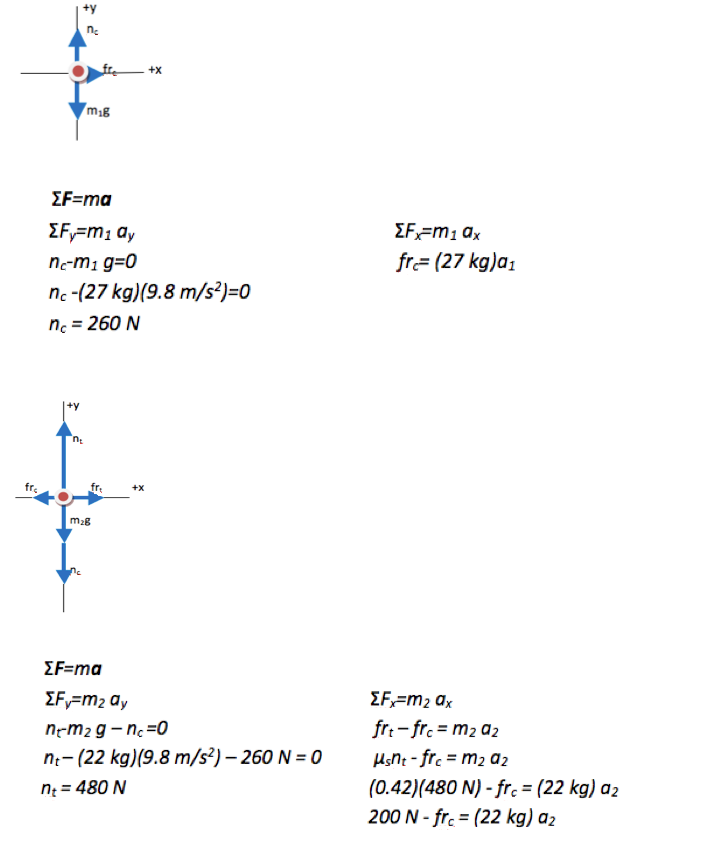One of the keys to successfully solving a multi-object problem algebraically is to keep track of the variables. I used different symbols for the masses of the two cartons because they are not the same, and I used different symbols for the accelerations of the cartons because we do not know if they move together or not. I used the same symbols for forces that are of equal strength (Third Law pairs.) If you did not recognize the Third Law pairs when you drew the FBDs, this would be the time to note and include that information.

Most two object problems would be algebraically solvable in this step. However, in this case we have three unknowns (a1, a2, and frc) and only two equations. Continue down to step 2 of this problem.

----------------------------------------------------------------------------------------------

Step 2

In this problem, we have three unknowns (a1, a2 and frc) and two equations: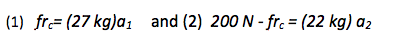We are asked to determine if the top carton stays on top of the bottom carton. We know conceptually that it does if the force of friction is great enough to provide the same acceleration that the bottom carton experiences. So the simplest solution is to assume that the boxes move together, in other words, that

a1 = a2 = a

and to compare the force of friction required to the maximum friction that is possible between the two boxes. When solving multiple equations in multiple unknowns, you can always solve one equation for one of the variables (I will solve equation (1) for a) and substitute it into the other equation.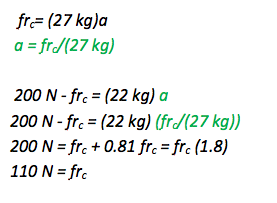So, again, it would require a frictional force of 110 N between the two cartons in order for the top carton to stay on top of the bottom one. The force of static friction between the two cartons is

frstatic ≤ µsn

frstatic ≤ (0.40)(260 N)

frstatic ≤ 100 N (so friction is not strong enough to keep the top carton on top of the bottom and it will slide off)

• In this problem, you were asked to consider the motion of two cartons.

The bottom carton experiences a backward force of friction with the floor of the truck (acting against its motion) and also a forward force of friction due to the upper carton sliding across it. Because the backward friction is greater than the forward friction, the bottom carton slows down and remains in place on the floor of the truck. The amount of friction with the floor is larger than the carton would experience if it did not have another box stacked on top of it. This is seen because nt is equal to the weight of the bottom carton plus the normal force of the upper carton pushing on it.

The top carton experiences only one force of friction, acting backwards across the surface with the lower carton. The maximum value for that force of friction is 100 N. It would require a force of 110 N to hold the top carton in place on top of the bottom carton, and so it will slide off.